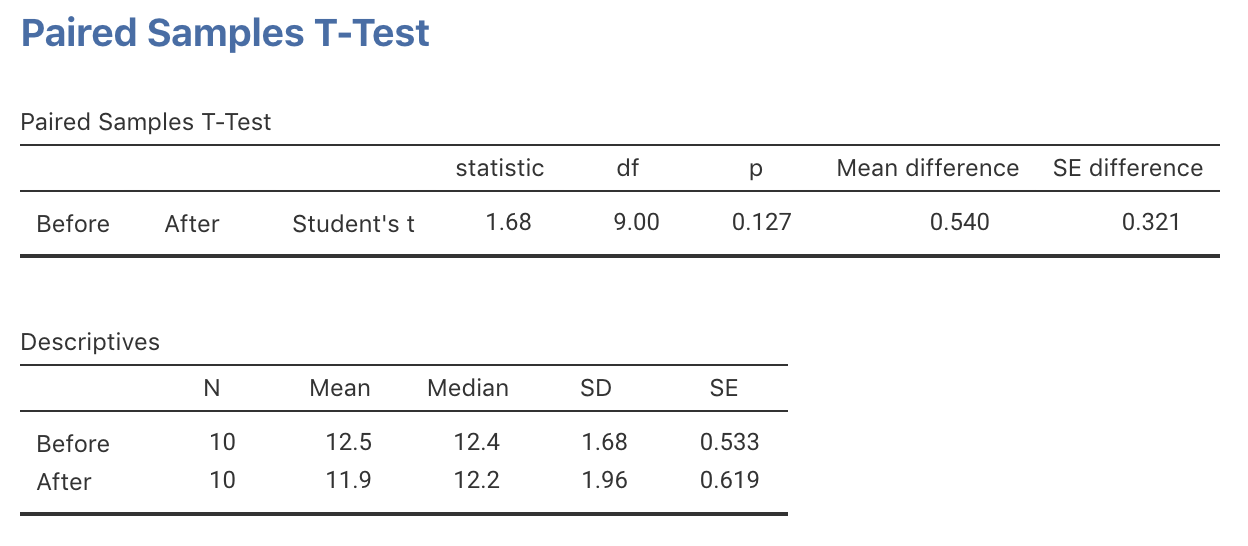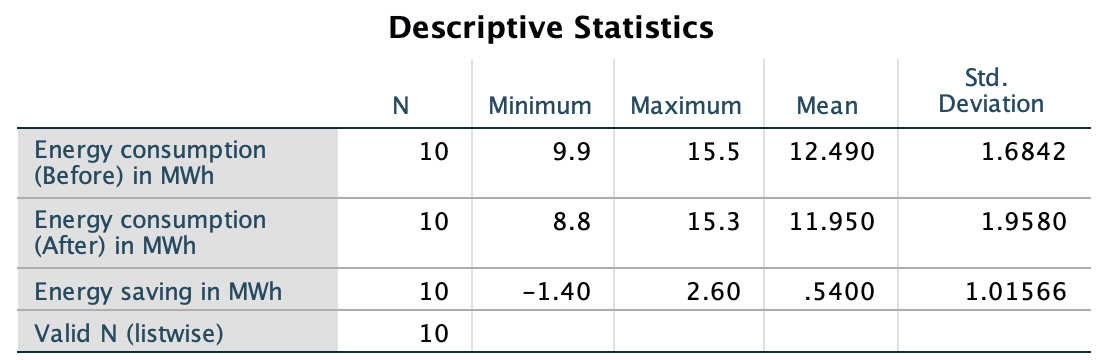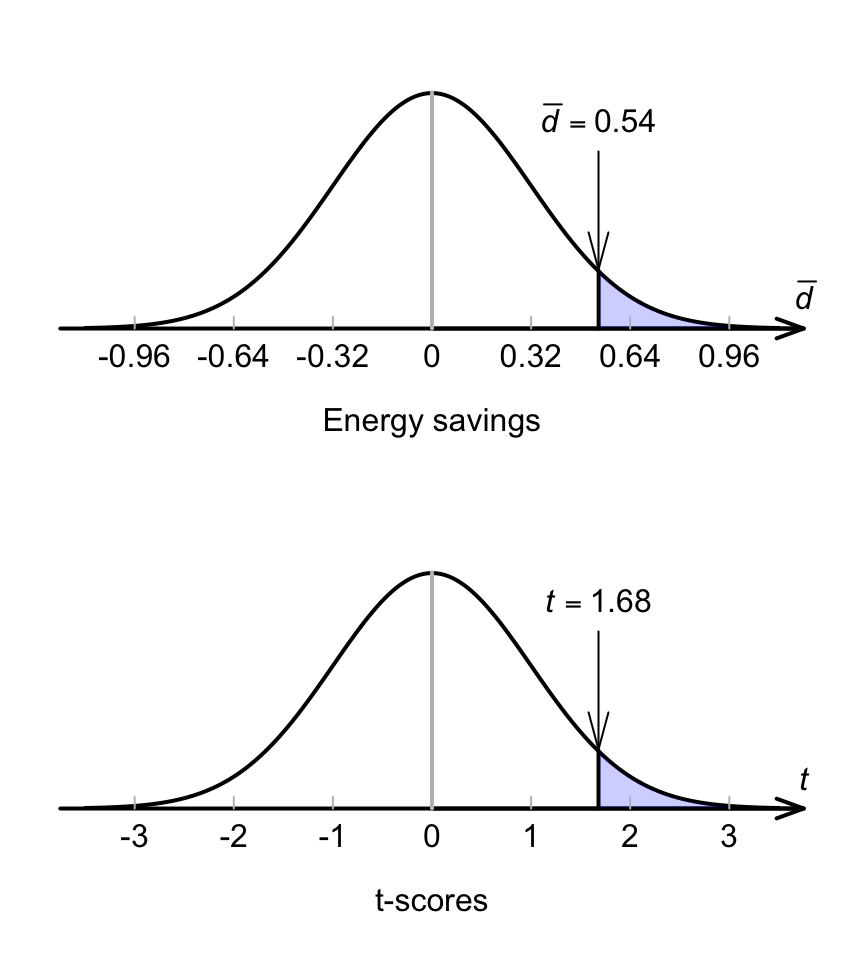## 29.3 Sampling distribution: Mean differences

Initially, assume that $$\mu_d = 0$$. However, the sample mean energy saving will vary depending on which sample is randomly obtained, even if the mean saving in the population is zero: the sample mean energy saving has sampling variation and hence a standard error. The sampling distribution of $$\bar{d}$$ can be described, which describes what values of the statistic might be expected in the sample if the $$\mu_d=0$$.

Answering the RQ requires data. The data should be summarised numerically (using your calculator or software, such as jamovi (Fig. 29.1) or SPSS (Fig. 29.2)), and graphically (Fig. 23.1).FIGURE 29.1: jamovi output for the insulation dataFIGURE 29.2: SPSS output for the insulation data

The sample mean difference is $$\bar{d}=0.5400$$… but this value of $$\bar{d}$$ will vary from sample to sample even if $$\mu_d=0$$. The amount of variation in $$\bar{d}$$ is quantified using the standard error. More precisely, the possible values of the sample mean differences can, under certain conditions, described using

• an approximate normal distribution; with
• a mean of $$\mu_d = 0$$ (taken from $$H_0$$); and
• a standard deviation (called the standard error) of $$\text{s.e.}(\bar{d}) = s_d/\sqrt{n} = 0.3212$$, where $$s_d$$ is the standard deviation of the differences.

This describes what can be expected from the possible values of $$\bar{d}$$ (Fig. 29.3), just through sampling variation (chance) if $$\mu_d=0$$.FIGURE 29.3: The sampling distribution of sample means, if the energy saving in the population really was zero